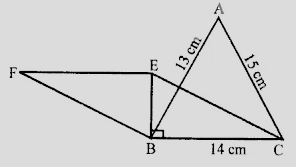# A triangle and a parallelogram have the same base and the same area. If the sides of the triangle are $13\ cm, 14\ cm$ and $15\ cm$ and the parallelogram stands on the base $14\ cm$, find the height of the parallelogram.

Given:

A triangle and a parallelogram have the same base and the same area.

The sides of the triangle are $13\ cm, 14\ cm$ and $15\ cm$ and the parallelogram stands on the base $14\ cm$.

To do:

We have to find the height of the parallelogram.

Solution:In $\triangle \mathrm{ABC}$,

$s=\frac{a+b+c}{2}$

$=\frac{13+14+15}{2}$

$=\frac{42}{2}$

$=21$

Area of the triangle $=\sqrt{s(s-a)(s-b)(s-c)}$

$=\sqrt{21(21-13)(21-14)(21-15)}$

$=\sqrt{21 \times 8 \times 7 \times 6}$

$=\sqrt{3 \times 7 \times 7 \times 3 \times 2 \times 2 \times 2 \times 2}$

$=3 \times 7 \times 2 \times 2$

$=84 \mathrm{~cm}^{2}$

Area of the parallelogram $=84 \mathrm{~cm}^{2}$

Base $\mathrm{BC}=14 \mathrm{~cm}$

This implies,

Height $=\frac{\text { Area }}{\text { base }}$

$=\frac{84}{14}$

$=6 \mathrm{~cm}$.

The height of the parallelogram is $6\ cm$.

Updated on: 10-Oct-2022

64 Views